# Truth-Functionally Complete Connectives

This is another “private communication” problem that I couldn’t find an obvious reduction for online.  I’ve used the holidays as an excuse to not put up a post so I could work on this problem, but even with the extra time, this problem has stumped me.

The problem: Truth-Functionally Complete Connectives.  This is problem LO10 in the appendix.

The description: Given a set U of variables, and a set C of well-formed Boolean expressions over U, is C truth-functionally complete?  In other words, can we find a finite set of unary and binary operators D = {θ1..θk} such that for each θi we can find an expression E in C, and a substitution s: U->{a,b} such that s(E) ≡ aθib (if θi is binary) or s(E) ≡ θia (if θi is unary)?

Example: So, “Truth-Functionally Complete” means that you can use the operations in D to generate all possible truth tables.  So some possible candidates for D are {AND, NOT} or just {NAND}.

So, if U = {x,y} and C = {~x, x∧y}, then I can just choose D to be {NOT, AND} and use the obvious substitution.  I think the hard part here is that the problem asks if such a D exists- I think the substitutions are probably always straightforward, once you get the functionally complete set D that gives you the best mapping..

Reduction: As I said above, this is a “Private Communication” problem, and so after a lot of searching, I found this paper by Statman, which I’m honestly am not even sure is the correct one. It at least talks about substitutions and deterministic polynomial time algorithms and things, but it very quickly goes into advanced Mathematical Logic that I wasn’t able to follow.  If someone out there knows more about this than I do and wants to chime in, let me know!

Edited Jan 22, 2020: Thanks to Thinh Nguyen for the help in coming up with the idea for the reduction- please check it out in the comments!

Difficulty: 10.  This is why we have the level 10 difficulty- for problems I can’t even follow.

### 10 responses to “Truth-Functionally Complete Connectives”

1.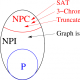Thinh D. Nguyen

For a set $E$ of Boolean wff (well-formed formulae) to be (truth table) functionally complete, two elementary formulae, namely $\lnot a$ and $a \land b$ needs to be substitution-realizable from $E$.

So, we reduce from 3-set Splitting which is NP-complete according to Wikipedia (see link below). To (always) satisfy the realization of $\lnot a$, we always include a separate formula $\lnot u$. That means the variable $u$ is not used elsewhere.

For each element in the given instance, we add a variable $x_i$.

Now, for each $3$-set $\{x_1,x_2,x_3\}$ (plz note that I do not want to write the complicated indices $i_1$, $i_2$, $i_3$), we create a sub-formula: $x_1\land x_2\land x_3$.

Then, we create a big formula by ORing all the above sub-formulae. Finally, output two formulae, namely the tiny one $\lnot u$ and the big-OR one.

A solution to the truth-functionally complete problem needs to “cut” every 3-set into a-part and b-part. And, vice versa.

https://en.wikipedia.org/wiki/Set_splitting_problem

2.Sean T. McCulloch

So, let me take this idea and flesh it out a little.
The reason we have a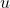that’s separate from any element of S (the set of Set Splitting variables) is so that we can set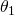to be. (And have it map to the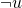expression).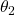will be “AND”. It will map to the giant expression (Basically forming a CNF-SAT instance of all of the sets we’re splitting). The formula will be equivalent to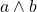if we can split the variables into “a” and “b” variables.

But what I’m confused about is the final formula. If we’re just OR-ing together all of the clauses,isn’t is possible that we can get an equivalent expression with an illegal split? (Just setting that clause to false, since if other clauses are true, the whole express will come out to true anyway..)

•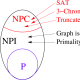Thinh D. Nguyen

“If we’re just OR-ing together all of the clauses,isn’t is possible that we can get an equivalent expression with an illegal split? (Just setting that clause to false, since if other clauses are true, the whole express will come out to true anyway..)”

But the point here is: “to find a substitution s: U->{a,b} such that s(E) ≡ aθib (if θi is binary)”

We are not reducing from 3-SAT.

3.Sean T. McCulloch

Right, so my question is:

Since we can take a true expression, and or it with anything else to still make a true expression, is it possible that there exist situations where we have an illegal split but still is logically equivalent to?

So, like, I did the truth table and things like (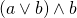) or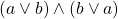aren’t equivalent. There’s no other way (without negations) to be equivalent, right?

4.Thinh D. Nguyen

An invalid split never yields any formula equivalent to $latex a \land b$.

As in your example, if we are given a NO instance which does not have any valid splid. Then if someone comes up with an invalid split which gives us the formula $latex (a\land b)\lor b$, then he/she just fails convincing us that the instance is YES one.

5.Sean T. McCulloch

Ok, I think I buy that.
Is it possible that in a NO instance we can come up with some _other_ set D of connectives that would work? (Since the problem only asks if one exists)

•Thinh D. Nguyen

Well, it is still possible to choose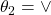instead of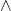.

But that is only possible when our given instance is disconnected into two connected components:

Only then, we can assign all literals in one connected component to a, the other connected component to b. Then (a AND a AND a) or (b AND b AND b) is just (a OR b).

But the NP-completeness proof assures us that the output instance is connected. We are safe.

•Sean T. McCulloch

But what if D had like 9 operations in it? There are all kinds of crazy sets of operations that over the entire set, form a complete set of operations.

Maybe we can avoid that by saying that since for each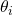we need to find an expression in E, and there are just 2 expressions in E, so any set D that has more than 2 elements is functionally equivalent to one with just 2 elements. And then we can show that there is just a small number of possible operations (exhaustively, if we have to), and show that for any set of 2 operations that’s complete, it has to split the variables.

Does that make sense?

•Thinh D. Nguyen

Yes, a detailed proof should be like that. And there are just a few cases to consider. Our second formula when substituted by a or b for each variable can only result in: a OR b, a AND b, (a AND b) OR b, (a AND b) OR a, (a AND b) OR a OR b, a, b. Not so many cases.

•Sean T. McCulloch

Cool- thanks for helping me out with this!